All UK Exam Boards included

The Hardy-Weinberg principle

How could we keep track of the frequency of each allele for a given trait when we have a dominant-recessive interaction? More specifically, how could we account for the visible dominant traits as homozygous or heterozygous, since both look the same?

This is where the Hardy-Weinberg principle comes in. Firstly, there are criteria for when this principle may be applied to a population:

1. Random mating must take place.
2. No migration must occur either inwards or outwards of the population.
3. No mutations must arise in the population.
4. No natural selection must take place due to one trait being better or worse adapted to the environment.

It’s apparent that this is simply rarely, if ever, the case in a real wild population. However, the Hardy-Weinberg principle is useful at predicting allele frequencies in a reliable mathematical model.

The frequency of the dominant allele is noted p while that of the recessive allele is noted q. Both must necessarily account for the whole population, therefore:

p + q = 1

The values are frequencies, so they are noted as percentages. 1 is 100% while 0.5 is 50% and 0.05 is 5%, etc.

Worked exercise

If we know that the frequency of the allele for dark fur in a population of koala bears is 0.2, and this allele is dominant over the one for light fur, work out the frequency of the allele for light fur in the population.

p = 0.2

p + q = 1

Therefore, 0.2 + q = 1 so q = 1 – 0.2

q = 0.8 or 80%.

Now the allele frequency has been worked out, how could we work out the actual phenotype of the koala bears in the population. How many are actually dark-furred? How many of the dark-furred ones are homozygous?

For this we use the same equation as before, but squared: (p + q)2

This is equivalent to p2 + 2pq + q2 = 1

Where 2pq is the frequency of heterozygotes, and p2 and q2 the frequencies of homozygous dominant and homozygous recessive respectively.

We want to know how many koala bears have dark fur. We know that the allele frequency for dark fur is 0.2, so 0.22 is the percentage of homozygous dark fur individuals; = 0.04 (4%).

This trait being dominant, the heterozygotes must also have dark fur. The frequency of heterozygous dark fur is 2pq = 2*0.2*0.8 = 0.32 (32%).

So overall, there are (0.4 + 0.32) 0.36 or 36% dark-furred koala bears in the population.

This leaves the remaining 64% with light fur. Note the contrast between the light phenotype only being 64% while the allele frequency for light fur is 80%. If the allele were dominant over dark fur, the frequency would be higher rather than lower.

Off-Spec Bonus Topics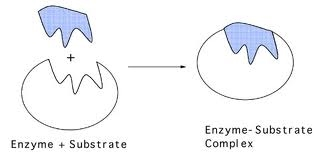1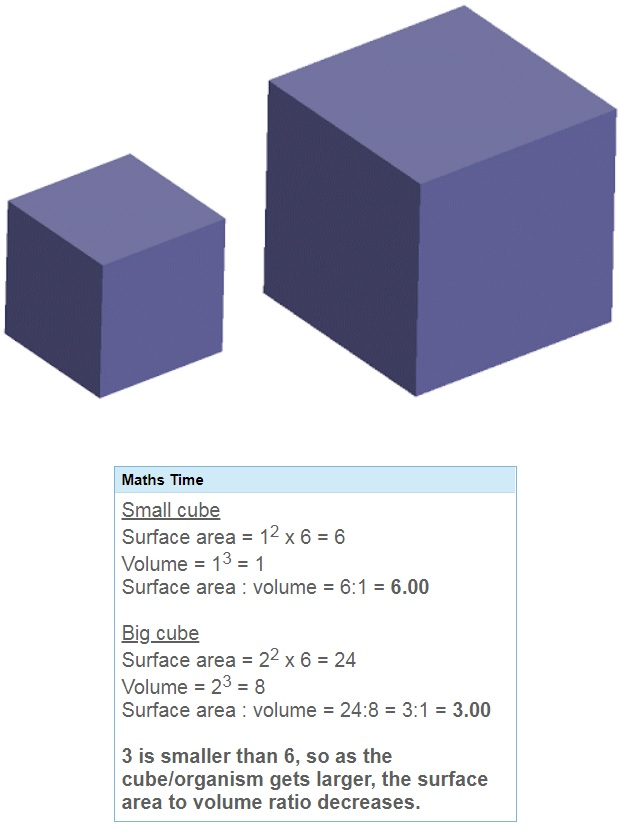1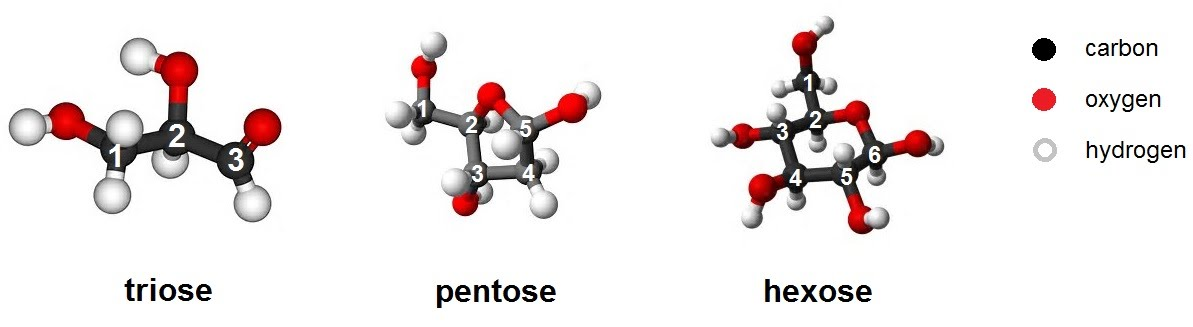1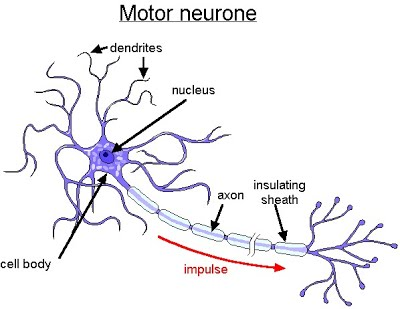1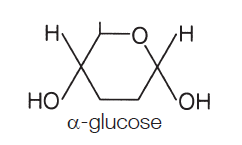1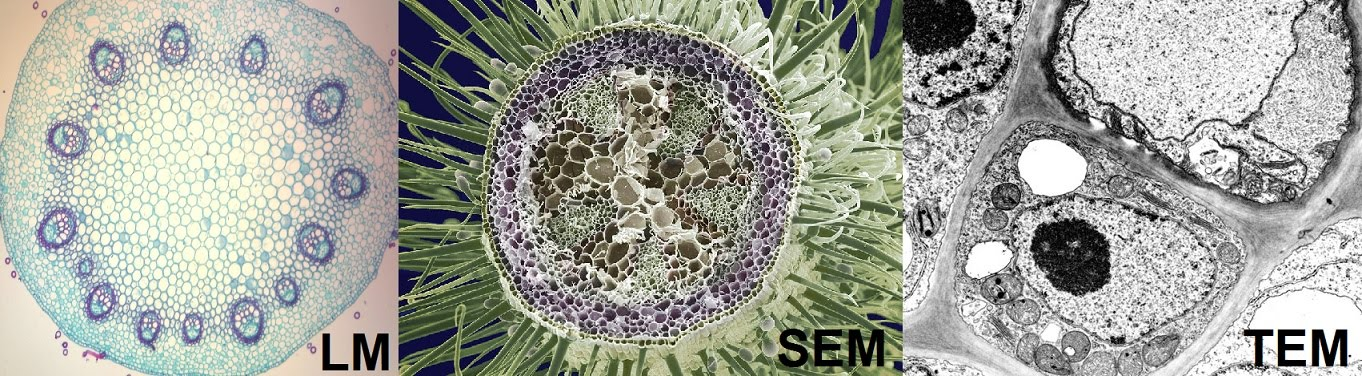1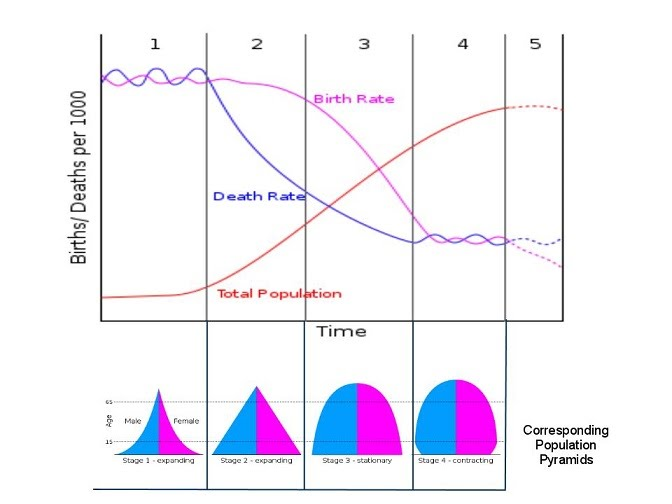11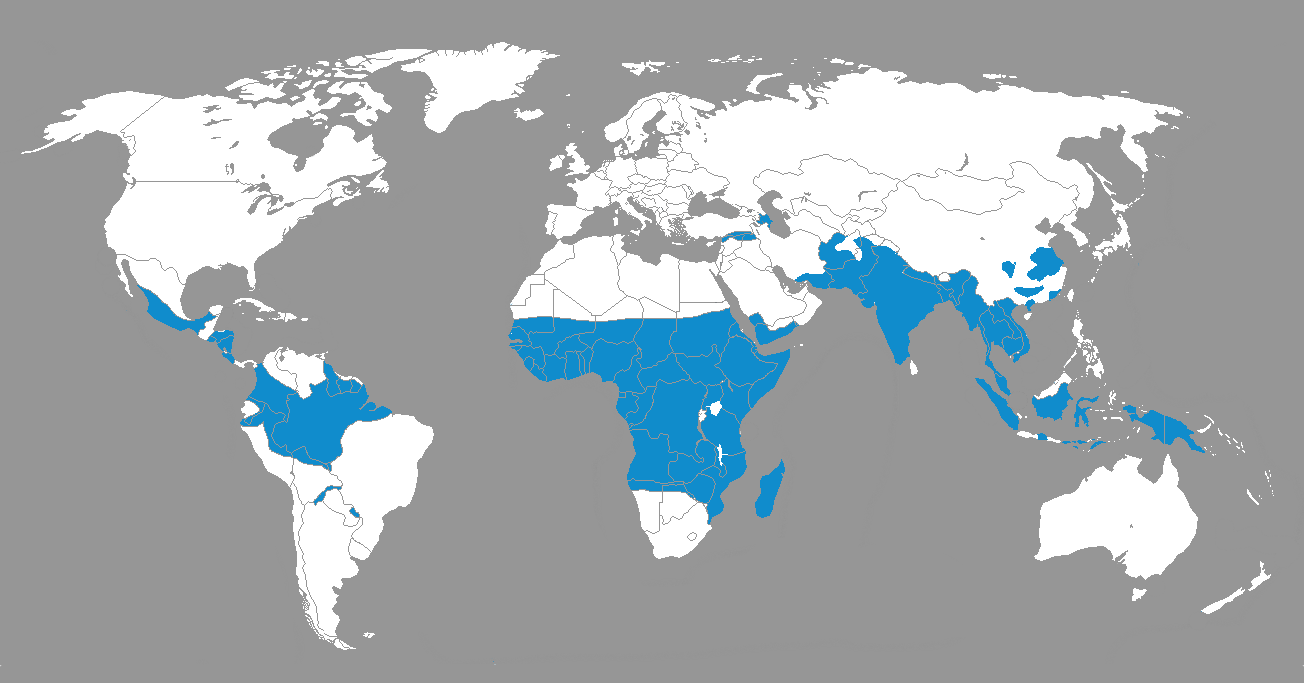11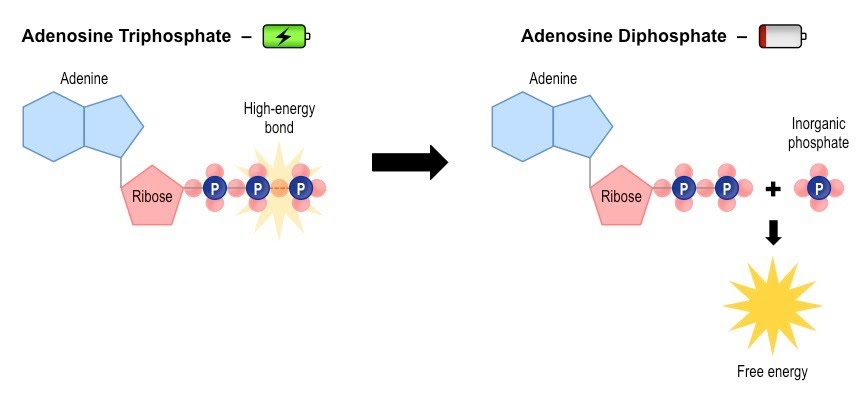1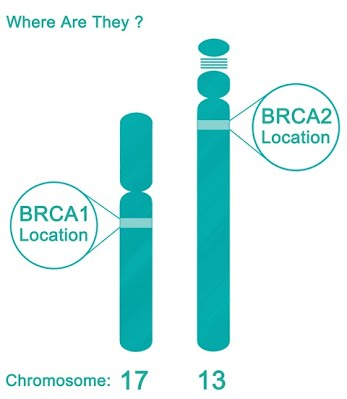1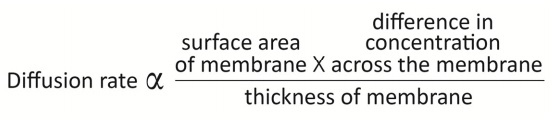11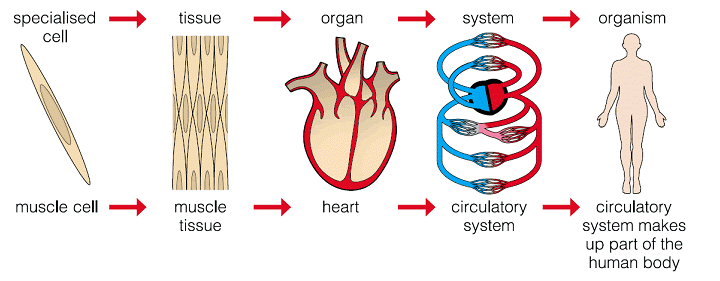111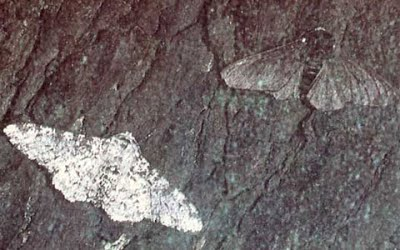1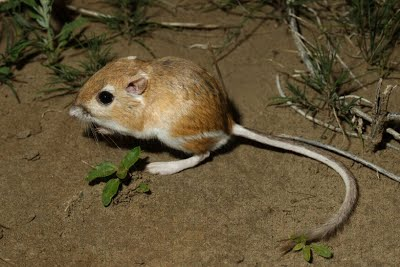111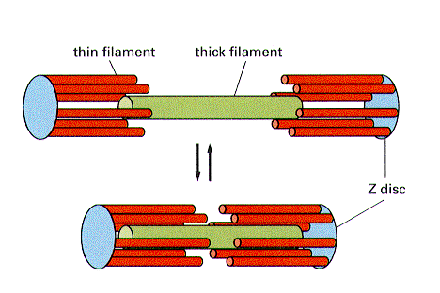11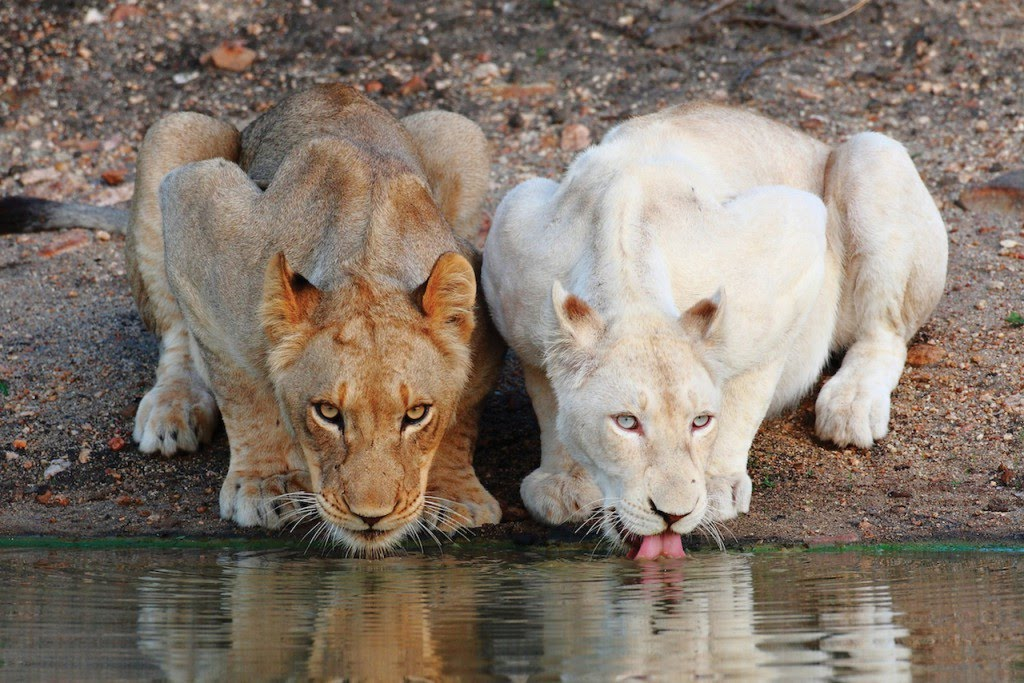11111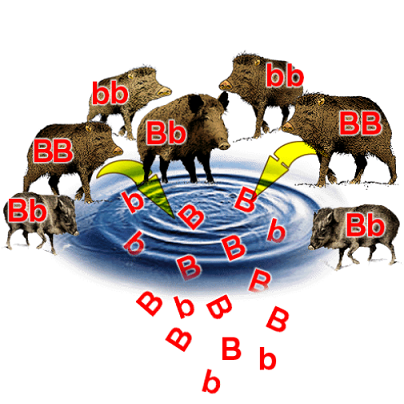1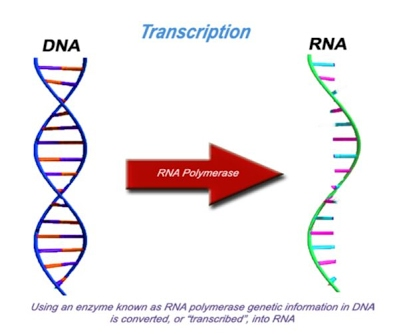1

Sorry! There are no posts.

Sorry! There are no posts.

I bookmarked the site

translucent The Student Room

OFF TOPIC : I just want to say that your website is brilliant, thank you!!